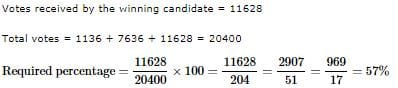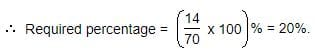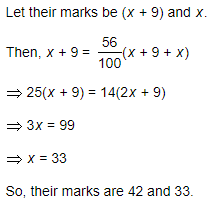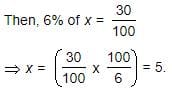Practice Test: Percentages- 2

# Practice Test: Percentages- 2

Test Description

## 10 Questions MCQ Test CSAT Preparation for UPSC CSE | Practice Test: Percentages- 2

Practice Test: Percentages- 2 for Quant 2023 is part of CSAT Preparation for UPSC CSE preparation. The Practice Test: Percentages- 2 questions and answers have been prepared according to the Quant exam syllabus.The Practice Test: Percentages- 2 MCQs are made for Quant 2023 Exam. Find important definitions, questions, notes, meanings, examples, exercises, MCQs and online tests for Practice Test: Percentages- 2 below.
Solutions of Practice Test: Percentages- 2 questions in English are available as part of our CSAT Preparation for UPSC CSE for Quant & Practice Test: Percentages- 2 solutions in Hindi for CSAT Preparation for UPSC CSE course. Download more important topics, notes, lectures and mock test series for Quant Exam by signing up for free. Attempt Practice Test: Percentages- 2 | 10 questions in 20 minutes | Mock test for Quant preparation | Free important questions MCQ to study CSAT Preparation for UPSC CSE for Quant Exam | Download free PDF with solutions
 1 Crore+ students have signed up on EduRev. Have you?
Practice Test: Percentages- 2 - Question 1

### If A = x% of y and B = y% of x, then which of the following is true?

Detailed Solution for Practice Test: Percentages- 2 - Question 1

x%ofy=x/100×y
=y/100×x
=y%ofx
∴A=B

Practice Test: Percentages- 2 - Question 2

### Three candidates contested an election and received 1136, 7636 and 11628 votes respectively. What percentage of the total votes did the winning candidate get?

Detailed Solution for Practice Test: Percentages- 2 - Question 2Practice Test: Percentages- 2 - Question 3

### What percentage of numbers from 1 to 70 have 1 or 9 in the unit's digit?

Detailed Solution for Practice Test: Percentages- 2 - Question 3

Clearly, the numbers which have 1 or 9 in the unit's digit, have squares that end in the digit 1. Such numbers from 1 to 70 are 1, 9, 11, 19, 21, 29, 31, 39, 41, 49, 51, 59, 61, 69.

Number of such number =14Practice Test: Percentages- 2 - Question 4

Two tailors X and Y are paid a total of Rs. 550 per week by their employer. If X is paid 120 percent of the sum paid to Y, how much is Y paid per week?

Detailed Solution for Practice Test: Percentages- 2 - Question 4

X+ y=Rs. 550
x = 120% of y
120% * y+ y =550
120/100*y+y = 550
6/5y+y =550
6y+5y/5 = 550
11/5y = 550
11y = 550*5
y = 250

Practice Test: Percentages- 2 - Question 5

Two numbers A and B are such that the sum of 5% of A and 4% of B is two-third of the sum of 6% of A and 8% of B. Find the ratio of A : B.

Detailed Solution for Practice Test: Percentages- 2 - Question 5

5100A+4100B=23[6A100+8B100]

5A+4B=2/3(6A+8B)

15A+12B=12A+16B

3A=4B

A/B=4/3⇒

A : B = 4 : 3

Practice Test: Percentages- 2 - Question 6

In an election between two candidates, one got 55% of the total valid votes, 20% of the votes were invalid. If the total number of votes was 7500, the number of valid votes that the other candidate got, was:

Detailed Solution for Practice Test: Percentages- 2 - Question 6

Total Valid votes are = 80% of 7500= 6000
If one has 55% vote then the other has left with 45% votes
Hence, 45% of 6000= 2700

Practice Test: Percentages- 2 - Question 7

Q. Two students appeared at an examination. One of them secured 9 marks more than the other and his marks was 56% of the sum of their marks. The marks obtained by them are:

Detailed Solution for Practice Test: Percentages- 2 - Question 7Practice Test: Percentages- 2 - Question 8

Gauri went to the stationers and bought things worth Rs. 25, out of which 30 paise went on sales tax on taxable purchases. If the tax rate was 6%, then what was the cost of the tax free items?

Detailed Solution for Practice Test: Percentages- 2 - Question 8

Let the amout taxable purchases be Rs. x.∴ Cost of tax free items = Rs. [25-(5+0.30)] = Rs. 19.70

Practice Test: Percentages- 2 - Question 9

A fruit seller had some apples. He sells 40% apples and still has 420 apples. Originally, he had:

Detailed Solution for Practice Test: Percentages- 2 - Question 9

x-x(40/100)=420
x-40x/100=420
or, 60x/100=420
x=42000/60
x= 700

Practice Test: Percentages- 2 - Question 10

If 20% of a = b, then b% of 20 is the same as:

Detailed Solution for Practice Test: Percentages- 2 - Question 10

20% of a = b  => (20/100)a = b

b% of 20 =(b/100) x 20 = (20a/100) x (1/100) x (20) = 4a/100 = 4% of a.

## CSAT Preparation for UPSC CSE

72 videos|64 docs|92 tests
 Use Code STAYHOME200 and get INR 200 additional OFF Use Coupon Code
Information about Practice Test: Percentages- 2 Page
In this test you can find the Exam questions for Practice Test: Percentages- 2 solved & explained in the simplest way possible. Besides giving Questions and answers for Practice Test: Percentages- 2, EduRev gives you an ample number of Online tests for practice

## CSAT Preparation for UPSC CSE

72 videos|64 docs|92 tests